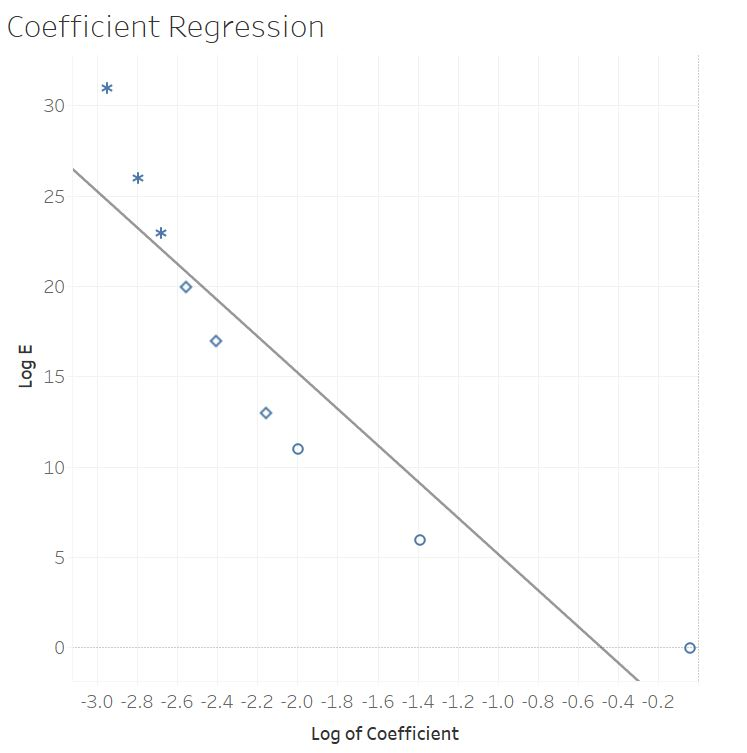# ToE

I recently wrote a post of quotes from Albert Einstein on my blog.  As I was researching, my curiosity was piqued regarding the Theory of Everything and how Data Science might be able to be applied to take a step to advance it.  I thought to myself that this would make an interesting thought exercise!

These quotes from Einstein particularly caught my attention:

• “Quantum mechanics is certainly interesting. But an inner voice tells me that it is not yet the real thing.  The theory says a lot, but does not really bring us any closer to the secret of the “old one”. I, at any rate, am convinced that He is not playing at dice”
• “I cannot seriously believe in it [quantum theory] because the theory cannot be reconciled with the idea that physics should represent a reality in time and space, free from spooky actions at a distance”
• “People like us, who believe in physics, know that the distinction between past, present and future is only a stubbornly persistent illusion”
• I believe in intuitions and inspirations. I sometimes feel that I am right. I do not know that I am.

And then I thought, maybe some principles from Data Science can help.  Here are some Data Science constructs that will help

• Logistic Regression
• Correlation Matrix
• Logarithmic Transformation
• Linear Regression

We start with our equations E = mc2 and E = hf (which is also E = hc/λ).  We know E = mc2 is in regards to large masses while E = hc/λ relates to infinitesimally small particles and waves.  This is a perfect case for Logistic Regression because these two equations are discrete classifications.  It is binary – either it is 1 or 0.  They also represent boundary conditions, so we can set each one to zero to solve them.  For the E = mc2 part of the equation, this stands alone and is correct as-is – for with large masses, we will have a value; for infinitesimally small masses this will go to zero.  For the E= hc/λ part of the equation, for infinitesimally small masses and waves, we will have a value; however, for large masses, we will have zero for a wave length so this will create a division by zero problem, so we will need a coefficient based on mass along with hf so that it can go to zero for large masses.  We will also have to apply a Logarithmic Transformation in order to analyze the data since it is skewed by magnitudes of size.  We can use a Correlation Matrix to test out different mathematical construct candidates and then finally, use a linear regression for the best candidate.

After some time exploring candidates, I came upon a promising candidate for the coefficient:  1/ ( (1-Log(mc2))^2).  I used  a small set of data in which I varied the mass from 1E-17 to 1E +14.  I plotted the logarithmic transformation of the coefficient in Tableau with R-squared of 0.85 and P value of 0.0004, which I think is pretty good on my hunt for a needle in the haystack.